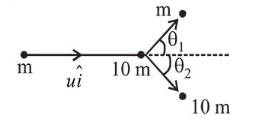# A particle of mass $m$ is moving alongQuestion:

A particle of mass $m$ is moving along the $x$-axis with initial velocity $u \hat{i}$. It collides elastically with a particle of mass 10 $\mathrm{m}$ at rest and then moves with half its initial kinetic energy

(see figure). If $\sin \theta_{1}=\sqrt{n} \sin \theta_{2}$, then value of $n$ is ______Solution:

From momentum conservation in perpendicular direction of initial motion.

$m u_{1} \sin \theta_{1}=10 m v_{1} \sin \theta_{2}$                       ...(1)

It is given that energy of $m$ reduced by half. If $u_{1}$ be velocity of $m$ after collision, then

$\left(\frac{1}{2} m u^{2}\right) \frac{1}{2}=\frac{1}{2} m u_{1}^{2}$

$\Rightarrow u_{1}=\frac{u}{\sqrt{2}}$

If $v_{1}$ be the velocity of mass $10 \mathrm{~m}$ after collision, then

$\frac{1}{2} \times 10 m \times v_{1}^{2}=\frac{1}{2^{m}} \frac{u^{2}}{2} \Rightarrow v_{1}=\frac{u}{\sqrt{20}}$

From equation (i), we have

$\sin \theta_{1}=\sqrt{10} \sin \theta_{2}$

$\Rightarrow n=10$